# 图像处理 霍夫变换

## 数字图像处理--霍夫变换

2018-09-18 10:22:46 hanjiangtao1995 阅读数 1431
• ###### 一学即懂的计算机视觉（第一季）

">图像处理和计算机视觉的课程大家已经看过很多，但总有“听不透”，“用不了”的感觉。课程致力于创建人人都能听的懂的计算机视觉，通过生动、细腻的讲解配合实战演练，让学生真正学懂、用会。</span> ...

32课时 312分钟 7017人学习 屈桢深
免费试看

# 霍夫变换概述

利用广义霍夫变换（GHT），找到模型位置的问题转换为寻找将模型映射到图像中的变换参数的问题。给定变换参数的值，就可以确定模型在图像中的位置。

# 霍夫变化原理

• 笛卡尔坐标系：可以由参数斜率和截距(m,b) 表示：
• 可由参数极径和极角(r,θ)表示：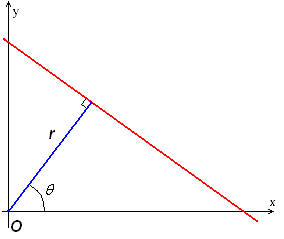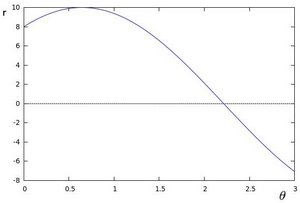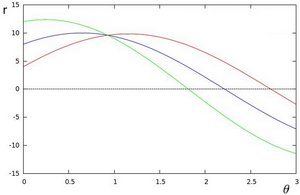# 霍夫圆变换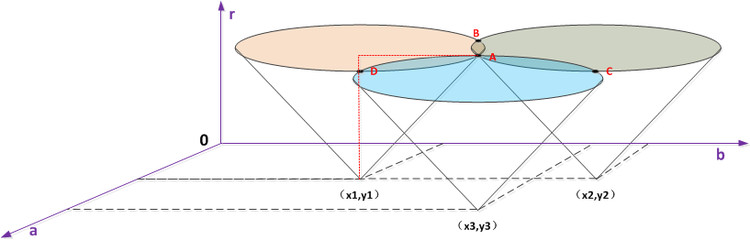## 图像处理之霍夫变换（直线检测算法）

2017-11-14 09:28:14 u010312937 阅读数 1806
• ###### 一学即懂的计算机视觉（第一季）

">图像处理和计算机视觉的课程大家已经看过很多，但总有“听不透”，“用不了”的感觉。课程致力于创建人人都能听的懂的计算机视觉，通过生动、细腻的讲解配合实战演练，让学生真正学懂、用会。</span> ...

32课时 312分钟 7017人学习 屈桢深
免费试看

X *cos(theta) + y * sin(theta)  = r 其中角度theta指r与X轴之间的夹角，r为到直线几何垂(x –a ) ^2 + (y-b) ^ 2 = r^2其中(a, b)为圆的中心点坐标，r圆的半径。这样霍夫的参数空间就

1.      读取一幅带处理二值图像，最好背景为黑色。

2.      取得源像素数据

3.      根据直线的霍夫变换公式完成霍夫变换，预览霍夫空间结果

4.       寻找最大霍夫值，设置阈值，反变换到图像RGB值空间(程序难点之一)

5.      越界处理，显示霍夫变换处理以后的图像

[java] view plain
1. // prepare for hough transform
2. int centerX = width / 2;
3. int centerY = height / 2;
4. double hough_interval = PI_VALUE/(double)hough_space;
5.
6. int max = Math.max(width, height);
7. int max_length = (int)(Math.sqrt(2.0D) * max);
8. hough_1d = new int[2 * hough_space * max_length];

[java] view plain
1. // start hough transform now....
2. int[][] image_2d = convert1Dto2D(inPixels);
3. for (int row = 0; row < height; row++) {
4.     for (int col = 0; col < width; col++) {
5.         int p = image_2d[row][col] & 0xff;
6.         if(p == 0continue// which means background color
7.
8.         // since we does not know the theta angle and r value,
9.         // we have to calculate all hough space for each pixel point
10.         // then we got the max possible theta and r pair.
11.         // r = x * cos(theta) + y * sin(theta)
12.         for(int cell=0; cell < hough_space; cell++ ) {
13.             max = (int)((col - centerX) * Math.cos(cell * hough_interval) + (row - centerY) * Math.sin(cell * hough_interval));
14.             max += max_length; // start from zero, not (-max_length)
15.             if (max < 0 || (max >= 2 * max_length)) {// make sure r did not out of scope[0, 2*max_lenght]
16.                 continue;
17.             }
18.             hough_2d[cell][max] +=1;
19.         }
20.     }
21. }

[java] view plain
1. // find the max hough value
2. int max_hough = 0;
3. for(int i=0; i<hough_space; i++) {
4.     for(int j=0; j<2*max_length; j++) {
5.         hough_1d[(i + j * hough_space)] = hough_2d[i][j];
6.         if(hough_2d[i][j] > max_hough) {
7.             max_hough = hough_2d[i][j];
8.         }
9.     }
10. }
11. System.out.println("MAX HOUGH VALUE = " + max_hough);
12.
13. // transfer back to image pixels space from hough parameter space
14. int hough_threshold = (int)(threshold * max_hough);

[java] view plain
1. // transfer back to image pixels space from hough parameter space
2. int hough_threshold = (int)(threshold * max_hough);
3. for(int row = 0; row < hough_space; row++) {
4.     for(int col = 0; col < 2*max_length; col++) {
5.         if(hough_2d[row][col] < hough_threshold) // discard it
6.             continue;
7.         int hough_value = hough_2d[row][col];
8.         boolean isLine = true;
9.         for(int i=-1; i<2; i++) {
10.             for(int j=-1; j<2; j++) {
11.                 if(i != 0 || j != 0) {
12.                   int yf = row + i;
13.                   int xf = col + j;
14.                   if(xf < 0continue;
15.                   if(xf < 2*max_length) {
16.                       if (yf < 0) {
17.                           yf += hough_space;
18.                       }
19.                       if (yf >= hough_space) {
20.                           yf -= hough_space;
21.                       }
22.                       if(hough_2d[yf][xf] <= hough_value) {
23.                           continue;
24.                       }
25.                       isLine = false;
26.                       break;
27.                   }
28.                 }
29.             }
30.         }
31.         if(!isLine) continue;
32.
33.         // transform back to pixel data now...
34.         double dy = Math.sin(row * hough_interval);
35.         double dx = Math.cos(row * hough_interval);
36.         if ((row <= hough_space / 4) || (row >= 3 * hough_space / 4)) {
37.             for (int subrow = 0; subrow < height; ++subrow) {
38.               int subcol = (int)((col - max_length - ((subrow - centerY) * dy)) / dx) + centerX;
39.               if ((subcol < width) && (subcol >= 0)) {
40.                   image_2d[subrow][subcol] = -16776961;
41.               }
42.             }
43.           } else {
44.             for (int subcol = 0; subcol < width; ++subcol) {
45.               int subrow = (int)((col - max_length - ((subcol - centerX) * dx)) / dy) + centerY;
46.               if ((subrow < height) && (subrow >= 0)) {
47.                   image_2d[subrow][subcol] = -16776961;
48.               }
49.             }
50.           }
51.     }
52. }[java] view plain
1. package com.gloomyfish.image.transform;
2.
3. import java.awt.image.BufferedImage;
4.
5. import com.process.blur.study.AbstractBufferedImageOp;
6.
7. public class HoughLineFilter extends AbstractBufferedImageOp {
8.     public final static double PI_VALUE = Math.PI;
9.     private int hough_space = 500;
10.     private int[] hough_1d;
11.     private int[][] hough_2d;
12.     private int width;
13.     private int height;
14.
15.     private float threshold;
16.     private float scale;
17.     private float offset;
18.
19.     public HoughLineFilter() {
20.         // default hough transform parameters
21.         //  scale = 1.0f;
22.         //  offset = 0.0f;
23.         threshold = 0.5f;
24.         scale = 1.0f;
25.         offset = 0.0f;
26.     }
27.
28.     public void setHoughSpace(int space) {
29.         this.hough_space = space;
30.     }
31.
32.     public float getThreshold() {
33.         return threshold;
34.     }
35.
36.     public void setThreshold(float threshold) {
37.         this.threshold = threshold;
38.     }
39.
40.     public float getScale() {
41.         return scale;
42.     }
43.
44.     public void setScale(float scale) {
45.         this.scale = scale;
46.     }
47.
48.     public float getOffset() {
49.         return offset;
50.     }
51.
52.     public void setOffset(float offset) {
53.         this.offset = offset;
54.     }
55.
56.     @Override
57.     public BufferedImage filter(BufferedImage src, BufferedImage dest) {
58.         width = src.getWidth();
59.         height = src.getHeight();
60.
61.         if ( dest == null )
62.             dest = createCompatibleDestImage( src, null );
63.
64.         int[] inPixels = new int[width*height];
65.         int[] outPixels = new int[width*height];
66.         getRGB( src, 00, width, height, inPixels );
67.         houghTransform(inPixels, outPixels);
68.         setRGB( dest, 00, width, height, outPixels );
69.         return dest;
70.     }
71.
72.     private void houghTransform(int[] inPixels, int[] outPixels) {
73.         // prepare for hough transform
74.         int centerX = width / 2;
75.         int centerY = height / 2;
76.         double hough_interval = PI_VALUE/(double)hough_space;
77.
78.         int max = Math.max(width, height);
79.         int max_length = (int)(Math.sqrt(2.0D) * max);
80.         hough_1d = new int[2 * hough_space * max_length];
81.
82.         // define temp hough 2D array and initialize the hough 2D
83.         hough_2d = new int[hough_space][2*max_length];
84.         for(int i=0; i<hough_space; i++) {
85.             for(int j=0; j<2*max_length; j++) {
86.                 hough_2d[i][j] = 0;
87.             }
88.         }
89.
90.         // start hough transform now....
91.         int[][] image_2d = convert1Dto2D(inPixels);
92.         for (int row = 0; row < height; row++) {
93.             for (int col = 0; col < width; col++) {
94.                 int p = image_2d[row][col] & 0xff;
95.                 if(p == 0continue// which means background color
96.
97.                 // since we does not know the theta angle and r value,
98.                 // we have to calculate all hough space for each pixel point
99.                 // then we got the max possible theta and r pair.
100.                 // r = x * cos(theta) + y * sin(theta)
101.                 for(int cell=0; cell < hough_space; cell++ ) {
102.                     max = (int)((col - centerX) * Math.cos(cell * hough_interval) + (row - centerY) * Math.sin(cell * hough_interval));
103.                     max += max_length; // start from zero, not (-max_length)
104.                     if (max < 0 || (max >= 2 * max_length)) {// make sure r did not out of scope[0, 2*max_lenght]
105.                         continue;
106.                     }
107.                     hough_2d[cell][max] +=1;
108.                 }
109.             }
110.         }
111.
112.         // find the max hough value
113.         int max_hough = 0;
114.         for(int i=0; i<hough_space; i++) {
115.             for(int j=0; j<2*max_length; j++) {
116.                 hough_1d[(i + j * hough_space)] = hough_2d[i][j];
117.                 if(hough_2d[i][j] > max_hough) {
118.                     max_hough = hough_2d[i][j];
119.                 }
120.             }
121.         }
122.         System.out.println("MAX HOUGH VALUE = " + max_hough);
123.
124.         // transfer back to image pixels space from hough parameter space
125.         int hough_threshold = (int)(threshold * max_hough);
126.         for(int row = 0; row < hough_space; row++) {
127.             for(int col = 0; col < 2*max_length; col++) {
128.                 if(hough_2d[row][col] < hough_threshold) // discard it
129.                     continue;
130.                 int hough_value = hough_2d[row][col];
131.                 boolean isLine = true;
132.                 for(int i=-1; i<2; i++) {
133.                     for(int j=-1; j<2; j++) {
134.                         if(i != 0 || j != 0) {
135.                           int yf = row + i;
136.                           int xf = col + j;
137.                           if(xf < 0continue;
138.                           if(xf < 2*max_length) {
139.                               if (yf < 0) {
140.                                   yf += hough_space;
141.                               }
142.                               if (yf >= hough_space) {
143.                                   yf -= hough_space;
144.                               }
145.                               if(hough_2d[yf][xf] <= hough_value) {
146.                                   continue;
147.                               }
148.                               isLine = false;
149.                               break;
150.                           }
151.                         }
152.                     }
153.                 }
154.                 if(!isLine) continue;
155.
156.                 // transform back to pixel data now...
157.                 double dy = Math.sin(row * hough_interval);
158.                 double dx = Math.cos(row * hough_interval);
159.                 if ((row <= hough_space / 4) || (row >= 3 * hough_space / 4)) {
160.                     for (int subrow = 0; subrow < height; ++subrow) {
161.                       int subcol = (int)((col - max_length - ((subrow - centerY) * dy)) / dx) + centerX;
162.                       if ((subcol < width) && (subcol >= 0)) {
163.                           image_2d[subrow][subcol] = -16776961;
164.                       }
165.                     }
166.                   } else {
167.                     for (int subcol = 0; subcol < width; ++subcol) {
168.                       int subrow = (int)((col - max_length - ((subcol - centerX) * dx)) / dy) + centerY;
169.                       if ((subrow < height) && (subrow >= 0)) {
170.                           image_2d[subrow][subcol] = -16776961;
171.                       }
172.                     }
173.                   }
174.             }
175.         }
176.
177.         // convert to hough 1D and return result
178.         for (int i = 0; i < this.hough_1d.length; i++)
179.         {
180.           int value = clamp((int)(scale * this.hough_1d[i] + offset)); // scale always equals 1
181.           this.hough_1d[i] = (0xFF000000 | value + (value << 16) + (value << 8));
182.         }
183.
184.         // convert to image 1D and return
185.         for (int row = 0; row < height; row++) {
186.             for (int col = 0; col < width; col++) {
187.                 outPixels[(col + row * width)] = image_2d[row][col];
188.             }
189.         }
190.     }
191.
192.     public BufferedImage getHoughImage() {
193.         BufferedImage houghImage = new BufferedImage(hough_2d.length, hough_space, BufferedImage.TYPE_4BYTE_ABGR);
194.         setRGB(houghImage, 00, hough_2d.length, hough_space, hough_1d);
195.         return houghImage;
196.     }
197.
198.     public static int clamp(int value) {
199.           if (value < 0)
200.               value = 0;
201.           else if (value > 255) {
202.               value = 255;
203.           }
204.           return value;
205.     }
206.
207.     private int[][] convert1Dto2D(int[] pixels) {
208.         int[][] image_2d = new int[height][width];
209.         int index = 0;
210.         for(int row = 0; row < height; row++) {
211.             for(int col = 0; col < width; col++) {
212.                 index = row * width + col;
213.                 image_2d[row][col] = pixels[index];
214.             }
215.         }
216.         return image_2d;
217.     }
218.
219. }

## 20180911图像处理之霍夫变换

2018-09-11 11:00:34 qq_18234121 阅读数 449
• ###### 一学即懂的计算机视觉（第一季）

">图像处理和计算机视觉的课程大家已经看过很多，但总有“听不透”，“用不了”的感觉。课程致力于创建人人都能听的懂的计算机视觉，通过生动、细腻的讲解配合实战演练，让学生真正学懂、用会。</span> ...

32课时 312分钟 7017人学习 屈桢深
免费试看

### 原理介绍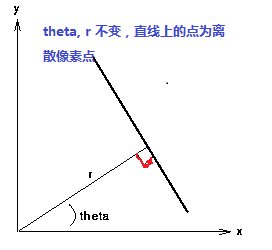让我们来看看霍夫变换是如何工作的。每一条直线都可以用(ρ; θ) 表示。所以首先创建一个2D 数组（累加器），初始化累加器，所有的值都为0。行表示ρ，列表示θ。这个数组的大小决定了最后结果的准确性。如果你希望角度精确到1 度，你就需要180 列。对于ρ，最大值为图片对角线的距离。所以如果精确度要达到一个像素的级别，行数就应该与图像对角线的距离相等。

想象一下我们有一个大小为100x100 的直线位于图像的中央。取直线上的第一个点，我们知道此处的（x，y）值。把x 和y 带入上边的方程组，然后遍历θ 的取值：0，1，2，3，...，180。分别求出与其对应的 的值，这样我们就得到一系列(ρ; θ) 的数值对，如果这个数值对在累加器中也存在相应的位置，就在这个位置上加1。所以现在累加器中的（50，90）=1。（一个点可能存在与多条直线中，所以对于直线上的每一个点可能是累加器中的多个值同时加1）。

### 编程思路解析：

1.      读取一幅带处理二值图像，最好背景为黑色。

2.      取得源像素数据

3.      根据直线的霍夫变换公式完成霍夫变换，预览霍夫空间结果

4.       寻找最大霍夫值，设置阈值，反变换到图像RGB值空间(程序难点之一)

5.      越界处理，显示霍夫变换处理以后的图像

## 图像处理基础知识系列之三：霍夫变换简单梳理

2017-05-31 19:40:02 coming_is_winter 阅读数 1166
• ###### 一学即懂的计算机视觉（第一季）

">图像处理和计算机视觉的课程大家已经看过很多，但总有“听不透”，“用不了”的感觉。课程致力于创建人人都能听的懂的计算机视觉，通过生动、细腻的讲解配合实战演练，让学生真正学懂、用会。</span> ...

32课时 312分钟 7017人学习 屈桢深
免费试看

# 霍夫变换之简单梳理

## 一、霍夫变换

霍夫变换(Hough Transform) 是图像处理中从图像中识别几何形状的基本方法之一，应用很广泛，也有很多改进算法。主要用来从图像中分离出具有某种相同特征的几何形状（如，直线，圆等）。最基本的霍夫变换是从黑白图像中检测直线(线段)。以上为百度百科内容。
霍夫变换另一个思路的理解是把一个空间内难以解决不好解决的问题转移到对应的其他空间寻求解决办法。现在应用到直线检测与圆的检测中。
先不涉及霍夫变换，先按普通的思路分析，怎样在一幅图片中找到存在其中的直线并把其画出来呢？其实对于人来讲比较简单，人眼搜索图片中所有区域，根据我们脑中直线的概念去找图片中对应的点的集合就对了。但是计算机不能这么做，我们看到的色彩绚烂的图片在计算机的理解中只是数量的矩阵，它能不能找到一条直线取决于根据人的参数设置找出对应的点的集合，而其自身没有对于直线的准确理解。

# 二、霍夫直线

在笛卡尔x,y坐标系下，已知一系列离散点，怎样找出一张直线呢？若下图所示：霍夫变换把xy坐标系下的方程转换为kb空间，把x,y当成常数，而k,b为变量，方程变为：b+xk=y,在xy空间的经过一个特定点的所有直线在k,b坐标系下就是一条直线（可以联系一下降维的概念，复杂问题简单化，霍夫变换的工作），举个例子：设特定点ct=(3,4)，在xy空间中由于k,b的取值不限，，所以经过特征点的直线集合为一个面。而在k,b空间，把ct带入b+xk=y后方程为b+3k=4,映射为一条直线，如下图所示：图2 左图为xy坐标系进过特定点ct的所有直线，未画完整      右图为所有直线在kb坐标系的投影，为一条直线看图2可以明白在xy坐标系下经过一个特征点的所有直线为一个面，映射到k,b空间为一条直线，看图3两个特定点映射到k,b空间为两条直线，两条直线相交确定一个点，这个交点（kt,bt）为在x,y的坐标系下表示经过两个特定点的一条直线(方程为kx+y=b）的kt,bt值。霍夫变换中还涉及到一个叠加原理，在这里表示为经过这个交点的直线越多，说明在xy坐标系下用kt,bt做参数的直线经过的点越多，这条直线越容易被检测出来。（以上图形为了直观展示，画图准确性不足）。现在我们回到计算量的问题上，图像上xy坐标系下的一个点映射到kb坐标系下画一条直线，计算一次，所以n个点对应着n次计算，计算量大幅减少，，霍夫变换的优势体现出来。
但由xy空间到kb空间有一个问题，看方程：kx+y=b，特定点确定后，当k=0时，b=y,当b=0时，k=y/x，通过这两个点 就可以把直线画出来了，特殊情况是x=0时，也就是有点在y轴上时，k=y/x除数为0，k确定不了，方程也确定不了了。
除了k,b空间，还有其他的空间吗？xy坐标系还可以映射到rθ坐标系，如图4左上图所示：y=(-cosθ/sinθ)x+(r/sinθ)，变换一下r=xcosθ+ysinθ。经过上边xy与kb空间变换我们得到的经验在这顺套，xy坐标系下的一条直线对应着rθ坐标系下的一个点，xy空间经过特定点的所有直线映射到到rθ空间为一条曲线（图4右上），多个特征点的所有直线映射到rθ空间为对应的曲线，若有交点，可以确定xy的一条直线（图4右下），同一交点上的曲线越多，说明xy空间一条直线上的已知点越多，直线越容易检测。同样计算量还是n级别的。解决了y轴点不能空间变换的问题。

## 三、霍夫圆

大体原理相似，圆在xy空间方程，（x-a)^2+(y-b)^2=r^2,映射到abr三维空间，方程为：（a-x）^2+(b-y)^2=r^2,设特定点为ct(xt,yt),则经过ct的所有圆映射到abr空间为r=sqrt((a-xt)^2+(b-yt)^2)。为一个圆锥面。如下图所示（特定点为（30,30））：两个特征点为两个圆锥面相交为一抛物线，三个圆锥面以上相交为一点，回到霍夫变换的叠加问题。
参考文献：

浅墨＿毛星云：http://blog.csdn.net/poem_qianmo/article/details/26977557/。

## 图像处理（八）——霍夫变换

2018-11-08 20:33:19 Godsolve 阅读数 355
• ###### 一学即懂的计算机视觉（第一季）

">图像处理和计算机视觉的课程大家已经看过很多，但总有“听不透”，“用不了”的感觉。课程致力于创建人人都能听的懂的计算机视觉，通过生动、细腻的讲解配合实战演练，让学生真正学懂、用会。</span> ...

32课时 312分钟 7017人学习 屈桢深
免费试看

#### 霍夫变换的过程可以分为以下几步：

1. 对输入图像进行边缘检测，获取边界点，即前景点。
2. 假如图像中存在圆形，那么其轮廓必定属于前景点（此时请忽略边缘提取的准确性）。
3. 同霍夫变换检测直线一样，将圆形的一般性方程换一种方式表示，进行坐标变换。由x-y坐标系转换到a-b坐标系。写成如下形式(a-x)²+(b-y)²=r²。那么x-y坐标系中圆形边界上的一点对应到a-b坐标系中即为一个圆。
4. 那x-y坐标系中一个圆形边界上有很多个点，对应到a-b坐标系中就会有很多个圆。由于原图像中这些点都在同一个圆形上，那么转换后a,b必定也满足a-b坐标系下的所有圆形的方程式。直观表现为这许多点对应的圆都会相交于一个点，那么这个交点就可能是圆心(a, b)。
5. 统计局部交点处圆的个数，取每一个局部最大值，就可以获得原图像中对应的圆形的圆心坐标(a,b)。一旦在某一个r下面检测到圆，那么r的值也就随之确定。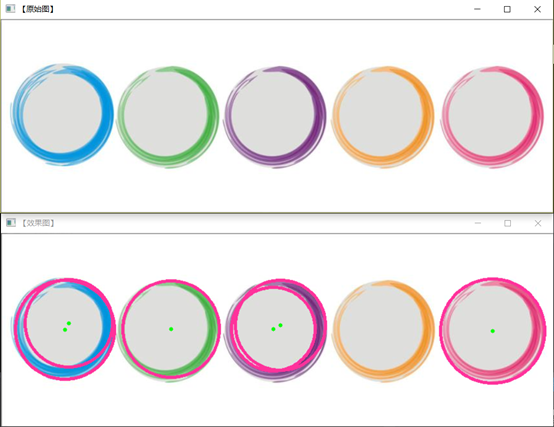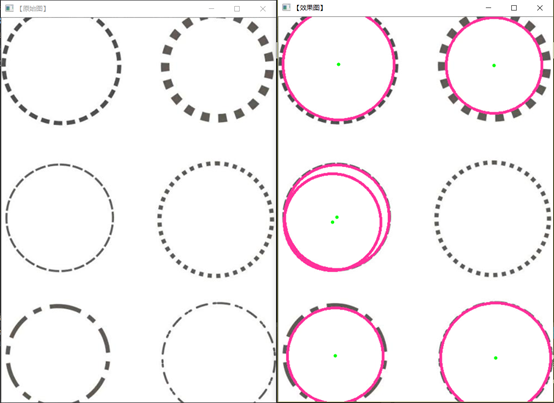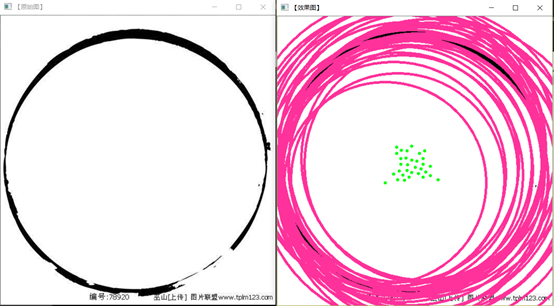##### 同时也欢迎各位关注我的微信公众号 南木的下午茶#include "stdafx.h"
#include "opencv2/core/core.hpp"
#include "opencv2/highgui/highgui.hpp"
#include "opencv2/imgproc/imgproc.hpp"
#include <opencv2/opencv.hpp>
#include <iostream>

using namespace std;
using namespace cv;

int main()
{
//【1】载入原始图和Mat变量定义
Mat midImage, dstImage;//临时变量和目标图的定义

//【2】显示原始图
imshow("【原始图】", srcImage);

//【3】转为灰度图，进行图像平滑
cvtColor(srcImage, midImage, CV_BGR2GRAY);//转化边缘检测后的图为灰度图
GaussianBlur(midImage, midImage, Size(9, 9), 2, 2);

//【4】进行霍夫圆变换
vector<Vec3f> circles;
HoughCircles(midImage, circles, CV_HOUGH_GRADIENT, 1.5, 10, 200, 100, 0, 0);

//【5】依次在图中绘制出圆
for (size_t i = 0; i < circles.size(); i++)
{
Point center(cvRound(circles[i]), cvRound(circles[i]));
//绘制圆心
circle(srcImage, center, 3, Scalar(0, 255, 0), -1, 8, 0);
//绘制圆轮廓
circle(srcImage, center, radius, Scalar(155, 50, 255), 3, 8, 0);
}

//【6】显示效果图
imshow("【效果图】", srcImage);

waitKey(0);

return 0;
}